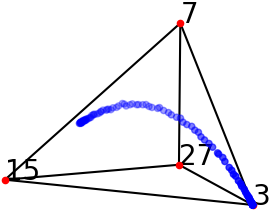# Lines on $$p$$-adic and real cubic surfaces

Rida Ait El Manssour, Yassine El Maazouz, Enis Kaya, and Kemal Rose: Lines on p-adic and real cubic surfaces
In: Abhandlungen aus dem Mathematischen Seminar der Universität Hamburg, Vol. not yet known, pp. not yet known

Abstract of the paper: We study lines on smooth cubic surfaces over the field of p-adic numbers, from a theoretical and computational point of view. Segre showed that the possible counts of such lines are 0,1,2,3,5,7,9,15 or 27. We show that each of these counts is achieved. Probabilistic aspects are also investigated by sampling both p-adic and real cubic surfaces from different distributions and estimating the probability of each count. We link this to recent results on probabilistic enumerative geometry. Some experimental results on the Galois groups attached to p-adic cubic surfaces are also discussed.In this repository you shall find the implementation of the numerical experiments in Table 3, Figure 1, and Section 5 of our paper.

The code we used to sample from different probability measures on 7-adic cubic surfaces can be viewed here:

The code we used to test the nonvanishing of any sum of 3 roots disscussed above Table 2 can be found here:

The code we used to sample real cubic surfaces can be found in the following Jupyter notebook:

To visualize the real cubic surfaces data, we used python. The code and data can be found in the following Jupyter notebook

System setup used for testing: MacBook Pro with macOS Monterey 12.0.1, Processor 3,3 GHz Intel Core i5, Memory 16 GB 2133 MHz LPDDR3, Graphics Intel Iris Graphics 550 1536 MB.

Computation were carried out using Linux clusters at MPI MiS and UC Berkeley.

Project page created: 02/04/2022.

Project contributors: Rida Ait El Manssour, Yassine El Maazouz, Enis Kaya, and Kemal Rose.

Software used: Magma (V2.26-10), Macaulay2, (verion 1.16) and Julia (version 1.7.1).